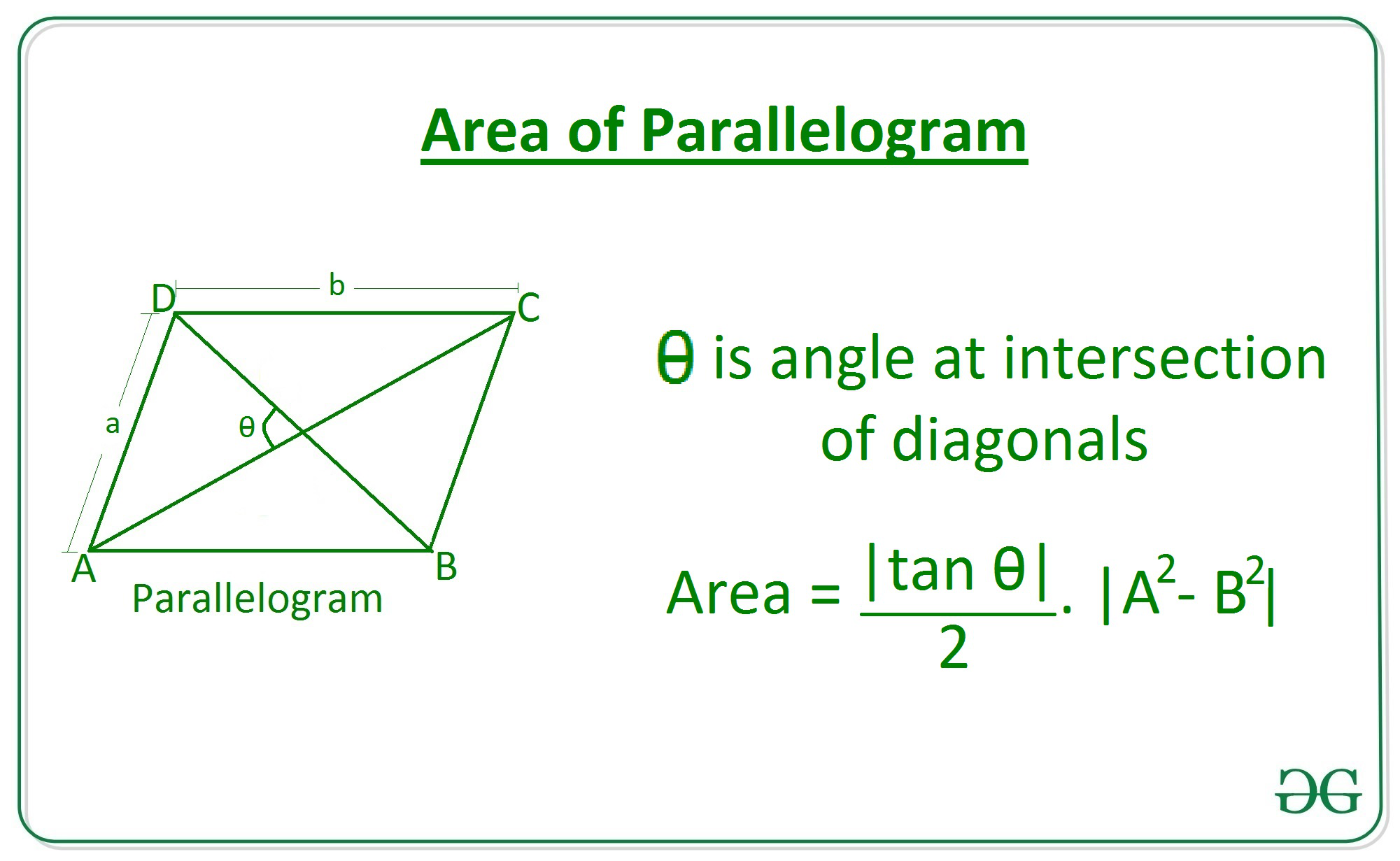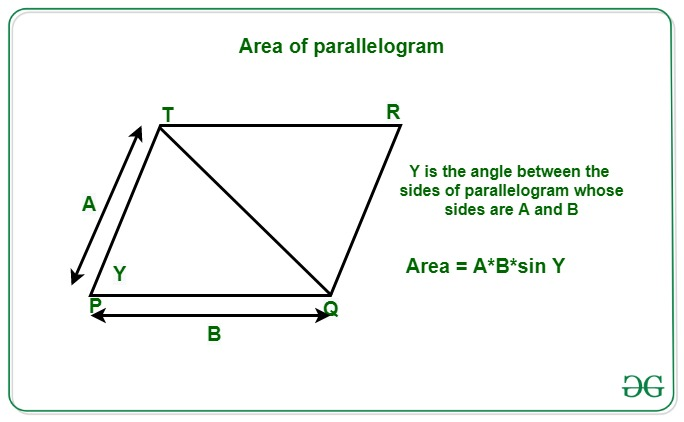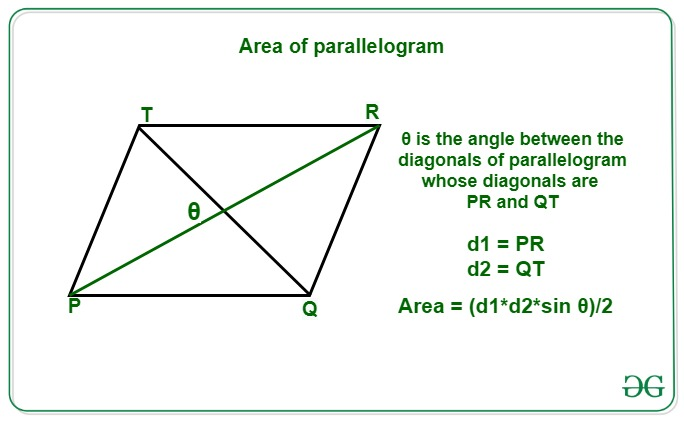# Program to calculate area of a parallelogram

• Last Updated : 05 Apr, 2021

Given integers A and B denoting the length of sides of a parallelogram and Y that is the angle between the sides and length of diagonals D1 and D2 of the parallelogram and an angle 0 at the intersection of the diagonal, the task is to find the area of the parallelogram from the information provided.

A parallelogram is a type of quadrilateral that has equal and parallel opposite sides and angle between them is not right angle.

Examples:

Input: A = 6, B = 10, 0 = 30
Output: 18.48
Explanation:
For the given sides 6 and 10 and for the angle 30 degree the area of parallelogram will be 18.48.

Input: A = 3, B = 5, Y = 45
Output: 10.61
Explanation:
For the given sides 3 and 5 and for the angle 45 degree the length of diagonal will be 10.61.

Input: D1 = 3, D2 = 5, 0 = 90
Output: 7.5
Explanation:
For the given diagonals 3 and 5 and for the angle 90 degree the area of parallelogram will be 7.5.

Approach: The area of the parallelogram can be calculated by the following three formulas:

• From given sides A and B and the angle between the diagonals, the area of the parallelogram can be calculated by the following formula:

Area of Parallelogram for sides and angle between diagonals = ((A2 – B2) * tan 0) / 2• From given sides A and B and the angle between the sides, the area of the parallelogram is can be calculated by the following formula:

Area of Parallelogram for sides and angle between sides = A * B * sin Y• From the given length of diagonals D1 and D2 and the angle between them, the area of the parallelogram can be calculated by the following formula:

Area of Parallelogram for diagonals and angle between diagonals = (D1 * D2 * sin 0)/2Below is the implementation of the above approach:

## C++

 `// C++ program for the``// above approach``#include ``using` `namespace` `std;` `double` `toRadians(``int` `degree)``{``  ``double` `pi = 3.14159265359;``  ``return` `((``double``)degree * (pi / 180));``}` `// Function to return the area of``// parallelogram using sides and``// angle at the intersection of diagonal``double` `Area_Parallelogram1(``int` `a, ``int` `b,``                           ``int` `theta)``{`` ` `    ``// Calculate area of parallelogram``    ``double` `area = (``abs``(``tan``(toRadians(theta))) / 2) *``                   ``abs``(a * a - b * b);`` ` `    ``// Return the answer``    ``return` `area;``}`` ` `// Function to return the area of``// parallelogram using sides and``// angle at the intersection of sides``double` `Area_Parallelogram2(``int` `a, ``int` `b,``                           ``int` `gamma)``{    ``  ``// Calculate area of parallelogram``  ``double` `area = (``abs``(``sin``(toRadians(gamma)))) *``                 ``abs``(a * b);` `  ``// Return the answer``  ``return` `area;``}`` ` `// Function to return the area of``// parallelogram using diagonals and``// angle at the intersection of diagonals``static` `double` `Area_Parallelogram3(``int` `d1, ``int` `d2,``                                  ``int` `theta)``{    ``  ``// Calculate area of parallelogram``  ``double` `area = (``abs``(``sin``(toRadians(theta))) / 2) *``                 ``abs``(d1 * d2);` `  ``// Return the answer``  ``return` `area;``}` ` ` `// Driver Code``int` `main()``{``  ``// Given diagonal and angle``  ``int` `d1 = 3;``  ``int` `d2 = 5;``  ``int` `theta = 90;` `  ``// Function call``  ``double` `area = Area_Parallelogram3(d1,``                                    ``d2,``                                    ``theta);``  ` `  ``// Print the area``  ``printf``(``"%.2f"``, area);``}` `// This code is contributed by rutvik_56`

## Java

 `// Java program for above approach``import` `java.io.*;` `class` `GFG{` `// Function to return the area of``// parallelogram using sides and``// angle at the intersection of diagonal``static` `double` `Area_Parallelogram1(``int` `a, ``int` `b,``                                  ``int` `theta)``{` `    ``// Calculate area of parallelogram``    ``double` `area = (Math.abs(Math.tan(``                   ``Math.toRadians(theta))) / ``2``) *``                   ``Math.abs(a * a - b * b);` `    ``// Return the answer``    ``return` `area;``}` `// Function to return the area of``// parallelogram using sides and``// angle at the intersection of sides``static` `double` `Area_Parallelogram2(``int` `a, ``int` `b,``                                  ``int` `gamma)``{``    ` `    ``// Calculate area of parallelogram``    ``double` `area = (Math.abs(Math.sin(``                   ``Math.toRadians(gamma)))) *``                   ``Math.abs(a * b);` `    ``// Return the answer``    ``return` `area;``}` `// Function to return the area of``// parallelogram using diagonals and``// angle at the intersection of diagonals``static` `double` `Area_Parallelogram3(``int` `d1, ``int` `d2,``                                  ``int` `theta)``{``    ` `    ``// Calculate area of parallelogram``    ``double` `area = (Math.abs(Math.sin(``                   ``Math.toRadians(theta))) / ``2``) *``                   ``Math.abs(d1 * d2);` `    ``// Return the answer``    ``return` `area;``}` `// Driver code``public` `static` `void` `main (String[] args)``{``    ` `    ``// Given diagonal and angle``    ``int` `d1 = ``3``;``    ``int` `d2 = ``5``;``    ``int` `theta = ``90``;``    ` `    ``// Function call``    ``double` `area = Area_Parallelogram3(``                  ``d1, d2, theta);``    ` `    ``// Print the area``    ``System.out.format(``"%.2f"``, area);``}``}` `// This code is contributed by offbeat`

## Python3

 `# Python3 program for the above approach` `import` `math` `# Function to return the area of``# parallelogram using sides and``# angle at the intersection of diagonal``def` `Area_Parallelogram1(a, b, theta):` `    ``# Calculate area of parallelogram``    ``area ``=` `(``abs``(math.tan(math.radians(theta)))``/``2``) \``           ``*` `abs``(a``*``*``2` `-` `b``*``*``2``)` `    ``# Return the answer``    ``return` `area` `# Function to return the area of``# parallelogram using sides and``# angle at the intersection of sides``def` `Area_Parallelogram2(a, b, gamma):` `    ``# Calculate area of parallelogram``    ``area ``=` `(``abs``(math.sin(math.radians(gamma)))) \``            ``*` `abs``(a ``*` `b)` `    ``# Return the answer``    ``return` `area` `# Function to return the area of``# parallelogram using diagonals and``# angle at the intersection of diagonals``def` `Area_Parallelogram3(d1, d2, theta):` `    ``# Calculate area of parallelogram``    ``area ``=` `(``abs``(math.sin(math.radians(theta)))``/``2``) \``            ``*` `abs``(d1 ``*` `d2)` `    ``# Return the answer``    ``return` `area`  `# Driver Code` `# Given diagonal and angle``d1 ``=` `3``d2 ``=` `5``theta ``=` `90` `# Function Call``area ``=` `Area_Parallelogram3(d1, d2, theta)``# Print the area``print``(``round``(area, ``2``))`

## C#

 `// C# program for``// the above approach``using` `System;``class` `GFG{` `// Function to return the area of``// parallelogram using sides and``// angle at the intersection of diagonal``static` `double` `Area_Parallelogram1(``int` `a, ``int` `b,``                                  ``int` `theta)``{``  ``// Calculate area of parallelogram``  ``double` `area = (Math.Abs(Math.Tan((theta *``                          ``Math.PI) / 180)) / 2) *``                 ``Math.Abs(a * a - b * b);` `  ``// Return the answer``  ``return` `area;``}` `// Function to return the area of``// parallelogram using sides and``// angle at the intersection of sides``static` `double` `Area_Parallelogram2(``int` `a, ``int` `b,``                                  ``int` `gamma)``{   ``  ``// Calculate area of parallelogram``  ``double` `area = (Math.Abs(Math.Sin((gamma *``                          ``Math.PI) / 180))) *``                 ``Math.Abs(a * b);` `  ``// Return the answer``  ``return` `area;``}` `// Function to return the area of``// parallelogram using diagonals and``// angle at the intersection of diagonals``static` `double` `Area_Parallelogram3(``int` `d1, ``int` `d2,``                                  ``int` `theta)``{   ``  ``// Calculate area of parallelogram``  ``double` `area = (Math.Abs(Math.Sin((theta *``                          ``Math.PI) / 180)) / 2) *``                 ``Math.Abs(d1 * d2);` `  ``// Return the answer``  ``return` `area;``}` `// Driver code``public` `static` `void` `Main(String[] args)``{``  ``// Given diagonal and angle``  ``int` `d1 = 3;``  ``int` `d2 = 5;``  ``int` `theta = 90;` `  ``// Function call``  ``double` `area = Area_Parallelogram3(d1, d2, theta);` `  ``// Print the area``  ``Console.Write(``"{0:F2}"``, area);``}``}` `// This code is contributed by Rajput-Ji`

## Javascript

 ``

Output:

`7.5`

Time Complexity: O(1)
Auxiliary Space: O(1)

My Personal Notes arrow_drop_up